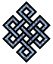fail2ban bad ip database: ip 117.40.138.21

| ip database | live view | stats | report | help | api key:

 ip: 117.40.138.21 hostname: 117.40.138.21 country:[CN] China first reported: 30.12.2017 04:13.45 GMT+0200 last reported: 24.03.2019 13:45.43 GMT+0200 time period: 449d 09h 31m 58s total reports: 4 reported by: 3 host(s) filter(s): ssh (3) ssh (1) tor exit node no badips.com db Lookupport scan of '117.40.138.21':

[-hide]
```# Nmap 6.40 scan initiated Sat Dec 30 04:14:02 2017 as: /usr/bin/nmap -sU -sS -O 117.40.138.21
Nmap scan report for 117.40.138.21
Host is up (0.27s latency).
Not shown: 1000 open|filtered ports, 987 closed ports
PORT      STATE    SERVICE
22/tcp    open     ssh
23/tcp    filtered telnet
80/tcp    open     http
135/tcp   filtered msrpc
139/tcp   filtered netbios-ssn
445/tcp   filtered microsoft-ds
593/tcp   filtered http-rpc-epmap
3306/tcp  open     mysql
4444/tcp  filtered krb524
5998/tcp  filtered ncd-diag
8443/tcp  open     https-alt
27000/tcp filtered flexlm0
54328/tcp filtered unknown
No exact OS matches for host (If you know what OS is running on it, see http://nmap.org/submit/ ).
TCP/IP fingerprint:
OS:SCAN(V=6.40%E=4%D=12/30%OT=22%CT=1%CU=%PV=N%G=Y%TM=5A4707A1%P=x86_64-pc-
OS:linux-gnu)SEQ(SP=F9%GCD=1%ISR=110%TI=I%TS=U)SEQ(SP=FE%GCD=1%ISR=110%TI=I
OS:%II=I%SS=S%TS=U)OPS(O1=M5B4%O2=M5B4%O3=M5B4%O4=M5B4%O5=M5B4%O6=M5B4)WIN(
OS:W1=2000%W2=2000%W3=2000%W4=2000%W5=2000%W6=2000)ECN(R=Y%DF=N%TG=FF%W=200
OS:0%O=M5B4%CC=N%Q=)ECN(R=N)T1(R=Y%DF=N%TG=FF%S=O%A=S+%F=AS%RD=0%Q=)T1(R=N)
OS:T2(R=N)T3(R=N)T4(R=N)T5(R=Y%DF=N%TG=FF%W=0%S=Z%A=S+%F=AR%O=%RD=0%Q=)T5(R
OS:=N)T6(R=N)T7(R=N)U1(R=N)IE(R=Y%DFI=S%TG=FF%CD=S)IE(R=N)

OS detection performed. Please report any incorrect results at http://nmap.org/submit/ .
# Nmap done at Sat Dec 30 04:27:29 2017 -- 1 IP address (1 host up) scanned in 807.33 seconds
```
```Σ = 69 | Δt = 0.0071830749511719s
```